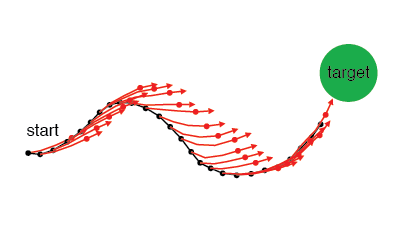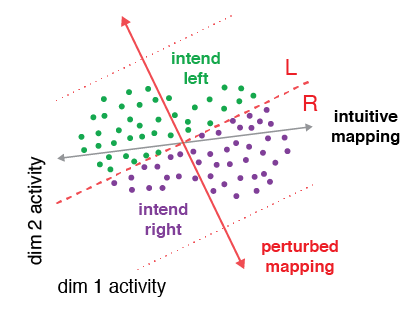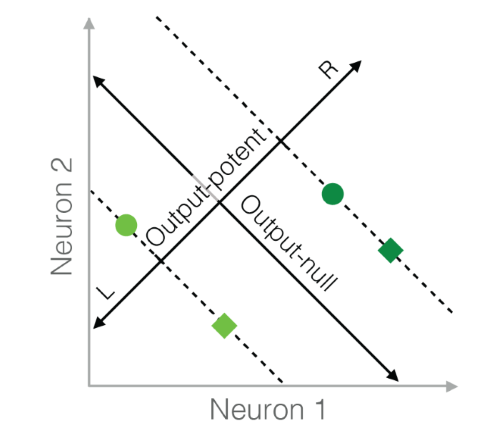# Software

## Internal Model Estimation (IME)IME estimates the subject's internal forward model of the relationship between the recorded neural activity and cursor movement. The inputs are the recorded neural population activity and the movement goal. The outputs are a linear forward model (model parameters), and an estimate at each time point of where the subject thinks the cursor is (latent variables).

## Neural ReassociationThis code implements five different hypotheses (Realignment, Rescaling, Reassociation, Partial Realignment, Subselection) of how neural population activity changes with learning. The inputs are the before-learning population activity, along with the BCI mappings. The outputs are predictions of after-learning population activity according to each hypothesis.

## Neural RedundancyThis code implements six different hypotheses of neural redundancy (Minimal Firing, Minimal Deviation, Uncontrolled-uniform, Uncontrolled-empirical, Persistent Strategy, and Fixed Distribution). The input is the activity in the output-potent space (i.e., behavior), and the output is the predicted distribution of neural activity in the output-null space according to each hypothesis.